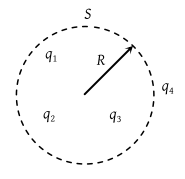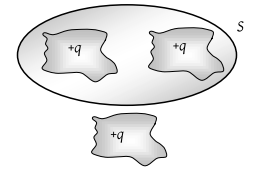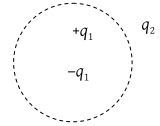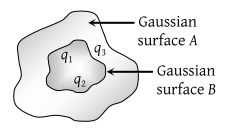q1, q2, q3 and q4 are point charges located at points as shown in the figure and S is a spherical Gaussian surface of radius R. Which of the following is true according to the Gauss’s law ?1. ${\oint }_{s}\left({\stackrel{\to }{E}}_{1}+{\stackrel{\to }{E}}_{2}+{\stackrel{\to }{E}}_{3}\right).d\stackrel{\to }{A}=\frac{{q}_{1}+{q}_{2}+{q}_{3}}{2{\epsilon }_{0}}$

2. ${\oint }_{s}\left({\stackrel{\to }{E}}_{1}+{\stackrel{\to }{E}}_{2}+{\stackrel{\to }{E}}_{3}\right).d\stackrel{\to }{A}=\frac{\left({q}_{1}+{q}_{2}+{q}_{3}\right)}{{\epsilon }_{0}}$

3. ${\oint }_{s}\left({\stackrel{\to }{E}}_{1}+{\stackrel{\to }{E}}_{2}+{\stackrel{\to }{E}}_{3}\right).d\stackrel{\to }{A}=\frac{\left({q}_{1}+{q}_{2}+{q}_{3}+{q}_{4}\right)}{{\epsilon }_{0}}$

4. None of the above

Concept Questions :-

Gauss law
High Yielding Test Series + Question Bank - NEET 2020

Difficulty Level:

If the electric flux entering and leaving an enclosed surface respectively is ${\phi }_{1}$ and ${\phi }_{2}$ the electric charge inside the surface will be

(1) $\left({\phi }_{1}+{\phi }_{2}\right){\epsilon }_{0}$

(2) $\left({\phi }_{2}-{\phi }_{1}\right){\epsilon }_{0}$

(3) $\left({\phi }_{1}+{\phi }_{2}\right)/{\epsilon }_{0}$

(4) $\left({\phi }_{2}-{\phi }_{1}\right)/{\epsilon }_{0}$

Concept Questions :-

Gauss law
High Yielding Test Series + Question Bank - NEET 2020

Difficulty Level:

Shown below is a distribution of charges. The flux of electric field due to these charges through the surface S is(1) $3q/{\epsilon }_{0}$

(2) $2q/{\epsilon }_{0}$

(3) $q/{\epsilon }_{0}$

(4) Zero

Concept Questions :-

Gauss law
High Yielding Test Series + Question Bank - NEET 2020

Difficulty Level:

Consider the charge configuration and spherical Gaussian surface as shown in the figure. When calculating the flux of the electric field over the spherical surface , the electric field will be due to(1) q2

(2) Only the positive charges

(3) All the charges

(4) +q1 and – q1

Concept Questions :-

Gauss law
High Yielding Test Series + Question Bank - NEET 2020

Difficulty Level:

An electric dipole is put in north-south direction in a sphere filled with water. Which statement is correct ?

(1) Electric flux is coming towards sphere

(2) Electric flux is coming out of sphere

(3) Electric flux entering into sphere and leaving the sphere are same

(4) Water does not permit electric flux to enter into sphere

High Yielding Test Series + Question Bank - NEET 2020

Difficulty Level:

The electric flux for Gaussian surface A that enclose the charged particles in free space is (given q1 = –14 nC, q2 = 78.85 nC, q3 = – 56 nC)(1) 103 Nm2 C–1

(2) 103 CN-1 m–2

(3) 6.32 × 103 Nm2 C–1

(4) 6.32 × 103 CN-1 m–2

Concept Questions :-

Gauss law
High Yielding Test Series + Question Bank - NEET 2020

Difficulty Level:

The electric intensity due to an infinite cylinder of radius R and having charge q per unit length at a distance r(r > R) from its axis is

(1) Directly proportional to r2

(2) Directly proportional to r3

(3) Inversely proportional to r

(4) Inversely proportional to r2

Concept Questions :-

Electric field
High Yielding Test Series + Question Bank - NEET 2020

Difficulty Level:

Two equal negative charge – q is fixed at the fixed points (0, a) and (0, –a) on the Y-axis. A positive charge Q is released from rest at the point (2a, 0) on the X-axis. The charge Q will

(1) Execute simple harmonic motion about the origin

(2) Move to the origin and remain at rest

(3) Move to infinity

(4) Execute oscillatory but not simple harmonic motion

Concept Questions :-

Electric field
High Yielding Test Series + Question Bank - NEET 2020

Difficulty Level:

An electric line of force in the xy plane is given by equation x2 + y2 = 1. A particle with unit positive charge, initially at rest at the point x = 1, y = 0 in the xy plane will -

(1) Not move at all

(2) Will move along straight line

(3) Will move along the circular line of force

(4) Information is insufficient to draw any conclusion

Concept Questions :-

Electric field
High Yielding Test Series + Question Bank - NEET 2020

Difficulty Level:

A positively charged ball hangs from a silk thread. We put a positive test charge q0 at a point and measure F/q0, then it can be predicted that the electric field strength E

(1) > F/q0

(2) = F/q0

(3) < F/q0

(4) Cannot be estimated

Concept Questions :-

Electric field